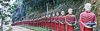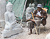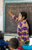# True Lazy Sequences25 Nov 2015

Lately I have read two very inspiring articles about sequences in Swift. Both articles use the sequence of Fibonacci numbers as an example of an unlimited and lazily evaluated sequence. In a lazy sequence its elements are not generated until their processing. However, there are some pitfalls that need to be avoided when working with such sequences.

The two articles, which I highly recommend reading, are## Custom Sequences

In general, a custom sequence can be defined by creating an instance of the `AnySequence` type which receives a function that creates a `GeneratorType` by using the `anyGenerator` function.If you don't know what a generator is, you should read one of the above articles first. For example the sequence of Fibonacci numbers can be defined as follows:

``````let fibs = AnySequence { () -> AnyGenerator<Int> in
var i = 0
var j = 1
return anyGenerator {
(i, j) = (j, i + j)
return i
}
}
``````

A simple `for` loop over this sequence will actually run infinitely

``````for f in fibs {
print(f)
}
``````

To output or reduce this sequence we have to limit it, for example by using the `prefix` function. Jacob's article uses `.prefix(7)` to compute the sum of the first 7 Fibonacci numbers:

``````fibs.prefix(7).reduce(0, combine: +) // returns 33
``````

Many use cases require computing a new sequence from a given sequence by applying the `filter` and `map` functions. In Colin's article (by now over a year old and using Swift 1) `filter` and `map` are free functions. However, these free functions are gone in Swift 2 and have been replaced by appropriate member functions of `SequenceType`. If we want to compute all even Fibonacci numbers, is seems obvious to replace this line of Swift 1 code:

``````let evenFibs = filter(fibs) { \$0 % 2 == 0}
``````

with this line in Swift 2:

``````let evenFibs = fibs.filter { \$0 % 2 == 0 }
``````

Unfortunately this doesn't work. XCode's playground (7.2 beta 3) even stops with a `EXC_BAD_INSTRUCTION`. So, what's wrong?

If we look at the definition of `filter` in `SequenceType` we find the following:

``````func filter(
@noescape includeElement: (Self.Generator.Element) throws -> Bool
) rethrows -> [Self.Generator.Element]
``````

Oops, this `filter` function returns an array! An array must always be computed completely; it is never lazy. That means the given filter `%0 % 2 == 0` will be evaluated eagerly on all elements of the sequence. Of course, this doesn't work for infinite sequences. The same is true for `map` and `flatMap`, these methods also return arrays. So what can we do about it?

The solution is to create an explicit lazy sequence by accessing the `lazy` property of a sequence:

``````let evenFibs = fibs.lazy.filter { \$0 % 2 == 0}
``````

By using `lazy` we get an instance of the `LazySequenceType` which offers lazy implementations of `filter`, `map`, and `flatMap`.This kind of lazy evaluation is not only important for infinite sequences, it may be useful for arrays as well. Whenever the manipulation of an array involves complex filter or map computations but we need only the first (or few) result elements then working on a lazy sequence will increase the overall performance by avoiding unnecessary computations.

I don't know why the lazily evaluated free functions `filter` and `map` of Swift 1 have been replaced by eagerly evaluated member functions in Swift 2. One benefit is of course that now we can turn every sequence (including arrays), no matter how it has been defined, into a lazily evaluated sequence.

However—as the following example demonstrates—you have to be careful not to switch back into eager mode when working with lazy sequences.

## FizzBuzz

Let's look at the FizzBuzz challenge. The task is to create a sequence of all natural numbers with the following exceptions:

• Every number that is divisible by 3 should be replaced with "Fizz".
• Every number that is divisible by 5 should be replaced with "Buzz".
• If both conditions are true then the number should be replaced with "FizzBuzz".The FizzBuzz sequence thus starts with: 1, 2, Fizz, 4, Buzz, Fizz, 7, 8, Fizz, Buzz, 11, Fizz, 13, 14, FizzBuzz, 16, 17, Fizz, 19, Buzz, Fizz, 22, 23, Fizz, Buzz, 26, Fizz, 28, 29, FizzBuzz, 31, ...

There are several imperative solutions to this problem that require thinking about the proper combination of modulo operations and the correct order of `if` statements.Apparently that's why the FizzBuzz challenge is sometimes used in job interviews for software developers. However, there is also a clean functional solution that goes as follows:The idea for this functional solution has been taken from the book Frege Goodness by Dierk König and Ingo Wechsung. Frege is definitely a language worth looking at, especially for developers on the JVM.

First: define a sequence of all numbers. This sequence is infinite, so let's turn it directly into a lazy typed sequence by adding `.lazy`

``````let numbers = AnySequence { () -> AnyGenerator<Int> in
var i = 1
return anyGenerator {
return i++
}
}.lazy
``````

Next: define two sequences of strings that have "Fizz" and "Buzz" as every third resp. fifth element and empty strings on all other positions:

``````let fizzes = numbers.map { \$0 % 3 == 0 ? "Fizz" : "" }
let buzzes = numbers.map { \$0 % 5 == 0 ? "Buzz" : "" }
``````

These `fizzes` and `buzzes` can be combined into a pattern sequence that consists of "Fizz", "Buzz", and "FizzBuzz" on their correct positions - simply by "zipping" both sequences and concatenating the element strings. `zip` is still a free function, so the expression for the pattern sequences is:

``````let pattern = zip(fizzes, buzzes)
.map { (fizz, buzz) in fizz + buzz }
``````

Do you think this is correct? No, it's not. The point is that `zip` creates a new sequence (of type `Zip2Sequence`) which provides again the eager `map` function (returning an array). Though the result of `zip` is in fact lazy, its type is not a lazy sequence type. To fix this we have to access the `lazy` property again:

``````let pattern = zip(fizzes, buzzes).lazy
.map { (fizz, buzz) in fizz + buzz }
``````

Finally we have to combine this `pattern` sequence with the original `numbers` sequence. If there is an empty pattern we have to use the number, otherwise we have to use the pattern. Again, this requires a combination of `zip` and `map`, and again we have to add `lazy`:

``````let fizzbuzz = zip(pattern, numbers).lazy
.map { (p, n) in p.isEmpty ? String(n) : p }
``````

(The full code is also available in this gist.)

In this example a missing `lazy` after using `zip` will be immediately detected because all sequences are infinite and an eager result of `map` can not be computed. However, if you start with an array, turn it into a lazy sequence and do some computations with it, take care not to fall back into the eager mode accidentally.

I'm interested in your feedback. Send me a mail or ping me on twitter.